# Math in Focus Grade 1 Cumulative Review Chapters 10 to 13 Answer Key

Practice the problems of Math in Focus Grade 1 Workbook Answer Key Cumulative Review Chapters 10 to 13 to score better marks in the exam.

## Math in Focus Grade 1 Cumulative Review Chapters 10 to 13 Answer Key

Concepts and Skills

Fill in the blanks.

Write heavier than, lighter than, or as heavy as.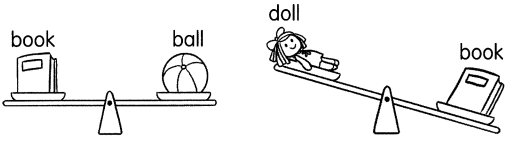Question 1.
The book is ___ the ball.
Answer: The book is heavier than the ball.

Question 2.
The doll is ___ the book.
Answer: The doll is lighter than the book.

Question 3.
The ball is ___ the doll.
Answer: The ball is heavier than the doll.

Fill in the blanks.

Question 4.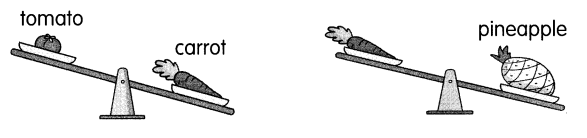The ____ is lighter than the carrot.
The ____ is heavier than the carrot.
So, the tomato is lighter than the pineapple.
The tomato is lighter than the carrot.
The pineapple is heavier than the carrot.

The picture graph shows the number of cars and trucks in a parking lot.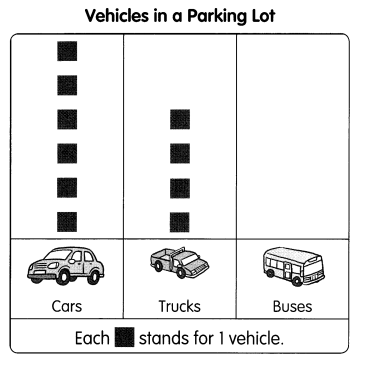Fill in the blanks.

Question 5.
There are ____ more cars than trucks.
Answer: There are 2 more cars than trucks.

Question 6.
There are ____ cars and trucks in all.
Answer: There are cars 10 and trucks in all.

Question 7.
There are 2 fewer buses than cars. Draw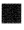to show the number of buses.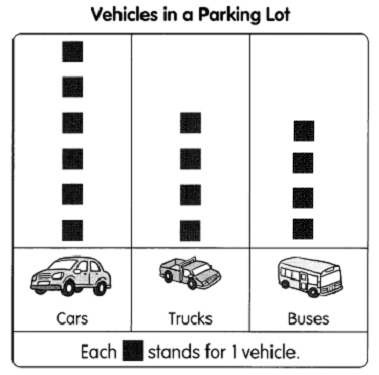Question 8.
Some cars leave the parking lot. The number of cars and trucks are now the same.
____ cars leave the parking lot.
Answer: 2 cars leave the parking lot.

Complete the tally chart using the data from the picture graph.

Question 9.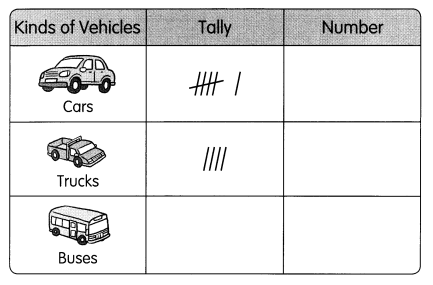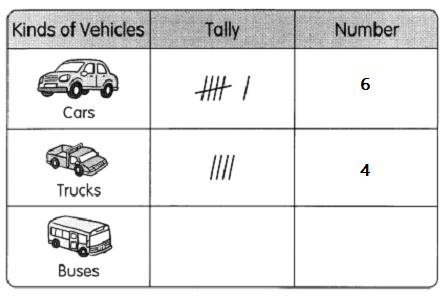Make a bar graph using the data from the tally chart.

Question 10.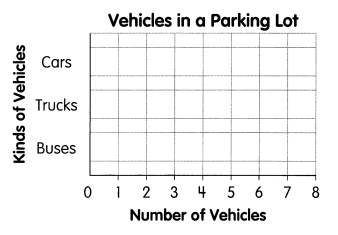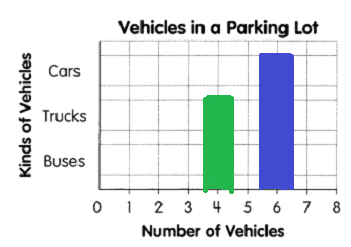Fill in the blanks.

1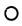stands for 1 unit.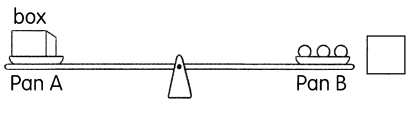Question 11.
The weight of the box is __ units.
Answer: The weight of the box is 3 units.

Question 12.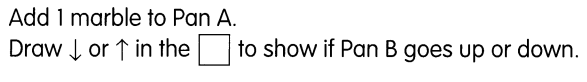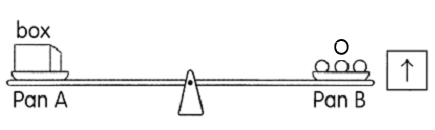Fill in the blanks.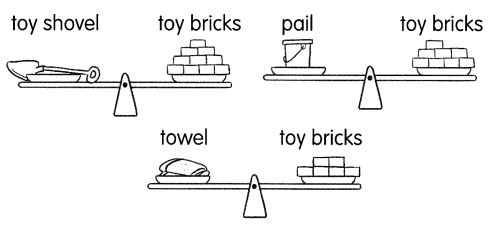Question 13.
The weight of the toy shovel is about ___ toy bricks.
Answer: The weight of the toy shovel is about 10 toy bricks.

Question 14.
Order the objects from heaviest to lightest.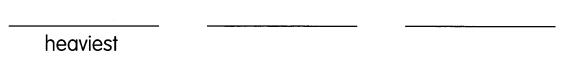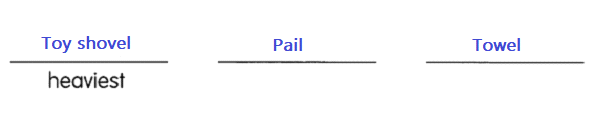First make tens.
Then count on.
Fill in the missing numbers.

Question 15.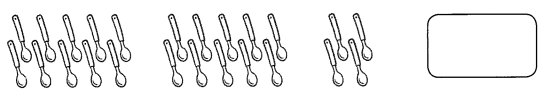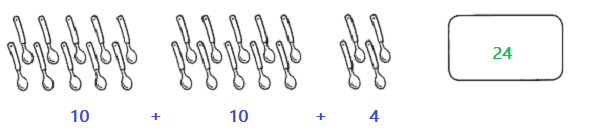Question 16.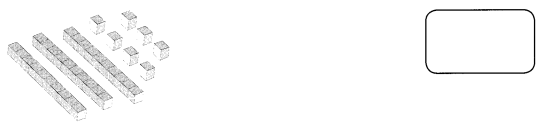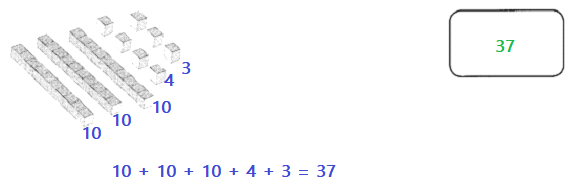Write the number.

Question 17.
twenty-five ____

Question 18.
thirty ____

Write the number in words.

Question 19.
37 _____

Question 20.
40 ____

Fill in the missing numbers.

Question 21.
1 + 20 = ____

Question 22.
___ + 10 = 36

Find the missing numbers.

Question 23.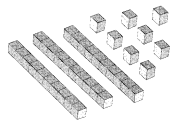38 = ___ tens ___ ones
Answer: 38 = 3 tens 8 ones

Look at the palce-value chart. Write the number it shows.

Question 24.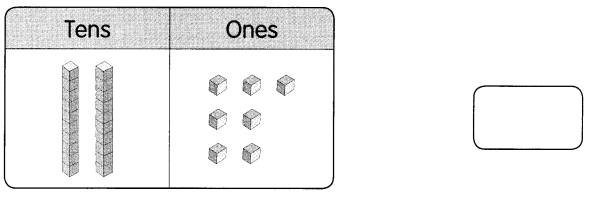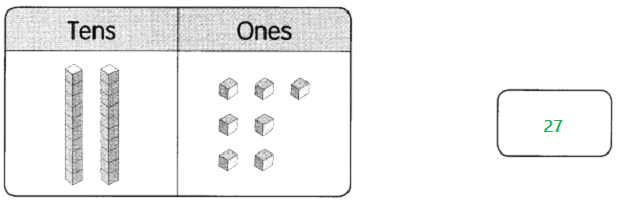Question 25.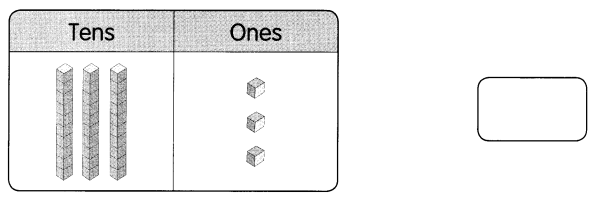Circle the greater number.

Question 26.
25 or 17
Answer: 25 is greater than 17

Question 27.
26 or 29
Answer: 29 is greater than 26

Circle the number that is less.

Question 28.
10 or 29

Question 29.
38 or 33

Compare the numbers. Then fill in the blanks.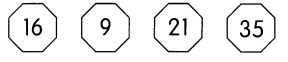Question 30.
___ is the least.

Question 31.
____ is the greatest.

Question 32.
____ is less than 35 but greater than 16
Answer: 21 is less than 35 but greater than 16

Question 33.
Order the numbers from the least to greatest.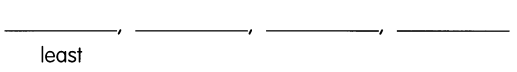Complete each number pattern.

Question 34.
17, 20, 23, ___, ____, 32
Answer: 17, 20, 23, 26 , 29, 32
Add 3 to each number to get the next number.

Question 35.
28, ___, 36, 40
Add 4 to each number to get the next number

Fill in the blanks.

Question 36.
2 less than 25 is ____

Question 37.
_______ is 3 more than 18.

Question 38.
24 = _________ tens 4 ones

Question 39.
18 = 1 ten _______ ones

Question 40.
21 + 7 = ____

Question 41.
24 + 10 = ___

Question 42.
27 – 3 = ____

Question 43.
38 – 15 = ____

Question 44.
6 + 3 + 7 = ____

Question 45.
9 + 8 + 5 = ___

Question 46.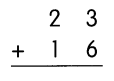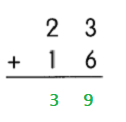Question 47.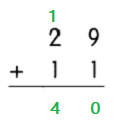Question 48.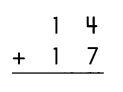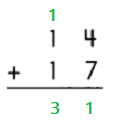Question 49.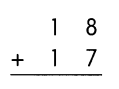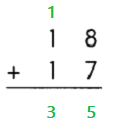Question 50.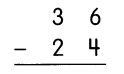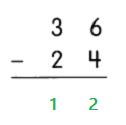Question 51.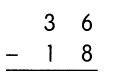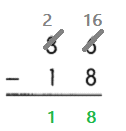Question 52.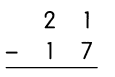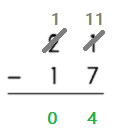Question 53.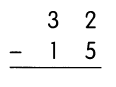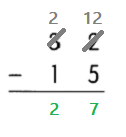Problem Solving

Solve.

Question 54.
Jamal has 20 stamps. Michelle has 4- fewer stamps than Jamal. How many stamps does Michelle have?
Michelle has ____ stamps.
Given,
Jamal has 20 stamps,
Michelle has 4 fewer stamps than Jamal,
By subtracting 4 from 20 we get 16,
Therefore, Michelle has 16 stamps.

Question 55.
Nate blows up 30 balloons for his birthday party. Nate blows up 6 fewer balloons than Miguel. How many balloons does Miguel blow up?
Miguel blows up ___ balloons.